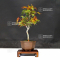The Japanese Bonsai specialist
Direct order Contact Help / Services NewsletterErable palmé "ori hime" 160702117

Acer palmatum ori himeref. : 11156

Product unavailable

Description

Height of the tree without its pot: 170 mm. Width of the branches: 130 mm. Enamelled pot of: 70*80 mm. Moyogi style. Ori hime species with tiny leaves, really adapted to the cultivation of small bonsaï. Photographed in May 2020. Shelf non-included.

#bonsai 3.6 #hime 3.4 #deciduous 2.9 #erable 2.6 #palme 2.5 #photographed 2.2 #cultivation 2.1 #enamelled 1.9 #branches 1.8 #included 1.8

Formule
(( ROUND((CHAR_LENGTH(b.article_nom)-CHAR_LENGTH(REPLACE(b.article_nom, 'hime', '')))/LENGTH('hime')) + ROUND((CHAR_LENGTH(b.article_description)-CHAR_LENGTH(REPLACE(b.article_description, 'hime', '')))/LENGTH('hime')) ) * 3.4) + (( ROUND((CHAR_LENGTH(b.article_nom)-CHAR_LENGTH(REPLACE(b.article_nom, 'erable', '')))/LENGTH('erable')) + ROUND((CHAR_LENGTH(b.article_description)-CHAR_LENGTH(REPLACE(b.article_description, 'erable', '')))/LENGTH('erable')) ) * 2.6) + (( ROUND((CHAR_LENGTH(b.article_nom)-CHAR_LENGTH(REPLACE(b.article_nom, 'palme', '')))/LENGTH('palme')) + ROUND((CHAR_LENGTH(b.article_description)-CHAR_LENGTH(REPLACE(b.article_description, 'palme', '')))/LENGTH('palme')) ) * 2.5) + (( ROUND((CHAR_LENGTH(b.article_nom)-CHAR_LENGTH(REPLACE(b.article_nom, 'photographed', '')))/LENGTH('photographed')) + ROUND((CHAR_LENGTH(b.article_description)-CHAR_LENGTH(REPLACE(b.article_description, 'photographed', '')))/LENGTH('photographed')) ) * 2.2) + (( ROUND((CHAR_LENGTH(b.article_nom)-CHAR_LENGTH(REPLACE(b.article_nom, 'cultivation', '')))/LENGTH('cultivation')) + ROUND((CHAR_LENGTH(b.article_description)-CHAR_LENGTH(REPLACE(b.article_description, 'cultivation', '')))/LENGTH('cultivation')) ) * 2.1) + (( ROUND((CHAR_LENGTH(b.article_nom)-CHAR_LENGTH(REPLACE(b.article_nom, 'enamelled', '')))/LENGTH('enamelled')) + ROUND((CHAR_LENGTH(b.article_description)-CHAR_LENGTH(REPLACE(b.article_description, 'enamelled', '')))/LENGTH('enamelled')) ) * 1.9) + (( ROUND((CHAR_LENGTH(b.article_nom)-CHAR_LENGTH(REPLACE(b.article_nom, 'branches', '')))/LENGTH('branches')) + ROUND((CHAR_LENGTH(b.article_description)-CHAR_LENGTH(REPLACE(b.article_description, 'branches', '')))/LENGTH('branches')) ) * 1.8) + (( ROUND((CHAR_LENGTH(b.article_nom)-CHAR_LENGTH(REPLACE(b.article_nom, 'included', '')))/LENGTH('included')) + ROUND((CHAR_LENGTH(b.article_description)-CHAR_LENGTH(REPLACE(b.article_description, 'included', '')))/LENGTH('included')) ) * 1.8) + (( ROUND((CHAR_LENGTH(b.article_nom)-CHAR_LENGTH(REPLACE(b.article_nom, 'without', '')))/LENGTH('without')) + ROUND((CHAR_LENGTH(b.article_description)-CHAR_LENGTH(REPLACE(b.article_description, 'without', '')))/LENGTH('without')) ) * 1.7) + (( ROUND((CHAR_LENGTH(b.article_nom)-CHAR_LENGTH(REPLACE(b.article_nom, 'adapted', '')))/LENGTH('adapted')) + ROUND((CHAR_LENGTH(b.article_description)-CHAR_LENGTH(REPLACE(b.article_description, 'adapted', '')))/LENGTH('adapted')) ) * 1.7)

Secure paymentDelivery

Our logistic partners :04 74 55 23 48
Pépinière MAILLOT-BONSAÏ
Le Bois Frazy
01990 RELEVANT - FRANCE
on appointment